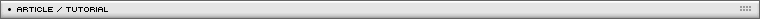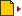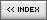3D perspective cube
[ July 22, 2004 ] by Eric Lin
Intermediate 3D scripting for Flash part 2/5:
Learn how to build a rotating cube in Flash 5 and also using the latest Flash MX drawing API

You can easily find tutorial about 3d "wire-framed" cube. Just setup 8 points in 3d space. Then apply the 3d equation to calculate the x,y of 2D, then connect them with lines. Now how to make "face" instead of  frames ?

In Flash MX, we can use the draw api to draw a filled face. In Flash 5, it is more complex. I use 3 skewed triangle to fill a face. So, I need 12 triangles.

Lets talk about the MX draw api first.

The first step is setting up the 8 points in 3d space.
The second step is setting up the 6 faces. Store the corners point to the face.

```clipArray=new Array();
clipArray.reg = [p0, p1, p2, p3];
// top
clipArray.reg = [p4, p5, p6, p7];
// bottom
clipArray.reg = [p0, p1, p5, p4];
// face 1
clipArray.reg = [p1, p2, p6, p5];
// face 2
clipArray.reg = [p2, p6, p7, p3];
// face 3
clipArray.reg = [p3, p7, p4, p0];
//face 4
```

Then in each face movieClip, I createEmptyMovieClip, and make it draw a filled quadrangle connecting the corners.

```beginFill(fColor, 100);
pt1 = f3dPoint(clip.reg);
pt2 = f3dPoint(clip.reg);
pt3 = f3dPoint(clip.reg);
pt4 = f3dPoint(clip.reg);
moveTo(pt1.x, pt1.y);
lineTo(pt2.x, pt2.y);
lineTo(pt3.x, pt3.y);
lineTo(pt4.x, pt4.y);
endFill();
```

Of course, I need 3D functions and rotation functions. They are described in other sessions. Well, in Flash 5, the clipArray will contain 12 clip elements. Each clip element is a triangle, so I store data to its reg array. The reg array will contain 3 elements instead of 4. Then make triangle skew to fill the triangle. The technique is similar to the movie "3d pyramid" that I describe in another sessions.